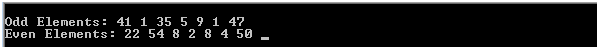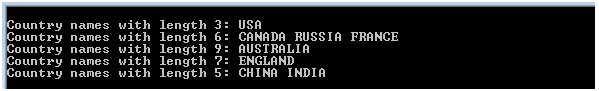# Understanding the LINQ GroupBy Operator in C#LINQ stands for Language Integrated Query and is Microsoft’s advanced data access technology that is used to query collections of data. The collections can be of two types: local collections such as collections belonging to the System.Collections namespace and the System.Collections.Generic spaces e.g. List<T>, Dictionary, HashSet etc. The other collections are remote collections such as an XML document or a database table. Most of the LINQ query operators can be implemented on both of these types of collections.  One such query operator is the GroupBy operator.

What is LINQ GroupBy Operator?

The LINQ GroupBy operator is different and unique in its own way in sense that it is the only operator that takes a flat sequence of items, organize that sequence into categories or groups and return groups of sequences. This is the only operator that falls in the category of grouping operator.

GroupBy operator does a task similar to what a librarian does while arranging new books on shelves. He checks the category or ISBN of a book and places it on the corresponding shelf. The category or the ISBN acts a key for grouping. The LINQ GroupBy operator performs a similar task; it also takes a collection of items as input, and then groups the items based on any particular property of the item, which is common between all the items. This concept is further explained with the help of an example.

A basic LINQ GroupBy example in C#

In the following example, the GroupBy operator takes a collection of random numbers and returns an IEnumerable collection of type IGrouping<key, element> where key is the type of key on the basis of which grouping is being done. The following example contains the source code:

```int[] numbers = { 41, 22, 54, 1, 8, 35, 5, 2, 9, 8, 4, 1, 47, 50 };

IEnumerable<IGrouping<bool, int>> groups = numbers.GroupBy(n => (n % 2 == 0));

foreach(IGrouping<bool,int> group in groups)

{

if (group.Key == true)

Console.Write("\nEven Elements: ");

else

Console.Write("\nOdd Elements: ");

foreach (int number in group)

{

Console.Write(number + " ");

}

}```

The code in the above example might look tricky at first but with little attention it can be easily comprehended. In the example, an integer type array named numbers has been instantiated with some random integers (both even and odd). The GroupBy operator then operates on the numbers collection.

The GroupBy key operator depends upon the return type of the lambda expression. In the example given above, the lambda expression “n => (n % 2 == 0)” would evaluate to true every time if the item ‘n’ mod 2 is zero, or in other words if ‘n’ is even. Therefore, a group of items is established that corresponds to true. Every time a number is even, the GroupBy operator’s key would be true.

Similarly, whenever the item ‘n’ in the lambda expression is odd, the key is false or the lambda expression in the GroupBy statement would evaluate to false. Another group of items is established where numbers are odd and the key is false. When all the elements are grouped in these two categories, the GroupBy statement returns the groups which can be stored in the IEnumerable collection of type IGrouping<key, element>. In the example above, this collection has been named ‘groups’.

Enumerating over the groups collection is a little bit tricky. First, every group should be enumerated via an outer foreach loop. Then inside the outer foreach loop, an inner foreach loop is used to enumerate over the elements in each particular group. In the example above, inside the outer foreach loop, a condition is checked that if the Key property of the group is true, display that this is an even group and then print its items, else display that this is an odd group and display its items. The console output of the code will look like this:Interested in learning more about C#? Take this course

Using LINQ GroupBy to Group String Elements by Length

The GroupBy operator can be used to group items in a string collection based on string length. The following example demonstrates how country names in the countries collection can be grouped based on the length of their names:

List<string> countries = new List<string> { “USA”, “CANADA”, “AUSTRALIA”, “ENGLAND”, “CHINA”,”INDIA” ,”RUSSIA”, “FRANCE” };

```IEnumerable<IGrouping<int, string>> lengthgroups = countries.GroupBy(n => n.Length);

foreach (IGrouping<int, string> lengthgroup in lengthgroups)

{

Console.Write("\nCountry names with length " + lengthgroup.Key + ": ");

foreach (string country in lengthgroup)

{

Console.Write(country + " ");

}

}```

In the above example, the type of the key for the Group by operator would be an integer, because grouping these items in the countries collection is being done based on the length of its names. Therefore, length is the key here and length is an integer type, therefore key would also be an integer type. The output of the above example looks like this:Using LINQ GroupBy with Custom Types

Like primitive types, the LINQ GroupBy operator can also be used to group a custom type object. Suppose there is class named Book which represents an actual book. It has a string variable name, an int variable ISBN and a string variable category. The Book class looks like this:

```class Book

{

public string name;

public int isbn;

public string category;

public Book (string name, int isbn, string category)

{

this.name = name;

this.isbn = isbn;

this.category = category;

}

}```

In the Main method, six objects of this class have been instantiated and added to the booklist List collection of type Book. This is done as follows:

```Book b1 = new Book("book1", 554654, "Mathematics");

Book b2 = new Book("book2", 454654, "English");

Book b3 = new Book("book3", 754654, "English");

Book b4 = new Book("book4", 854654, "History");

Book b5 = new Book("book5", 154654, "Mathematics");

Book b6 = new Book("book6", 354654, "History");

List<Book> booklist = new List<Book> { b1, b2, b3, b4, b5, b6 };```

There are six books and three categories: Mathematics, English and History. This booklist collection can be grouped into a group of books using the book category as the key as follows:

```IEnumerable<IGrouping<string, Book>> booklistgroups = booklist.GroupBy(b => b.category);

foreach (IGrouping<string, Book> bookgroup in booklistgroups)

{

Console.Write("\nBooks in " + bookgroup.Key +" category: ");

foreach (Book book in bookgroup)

{

Console.Write(book.name+" ");

}

}```

The output looks like this: